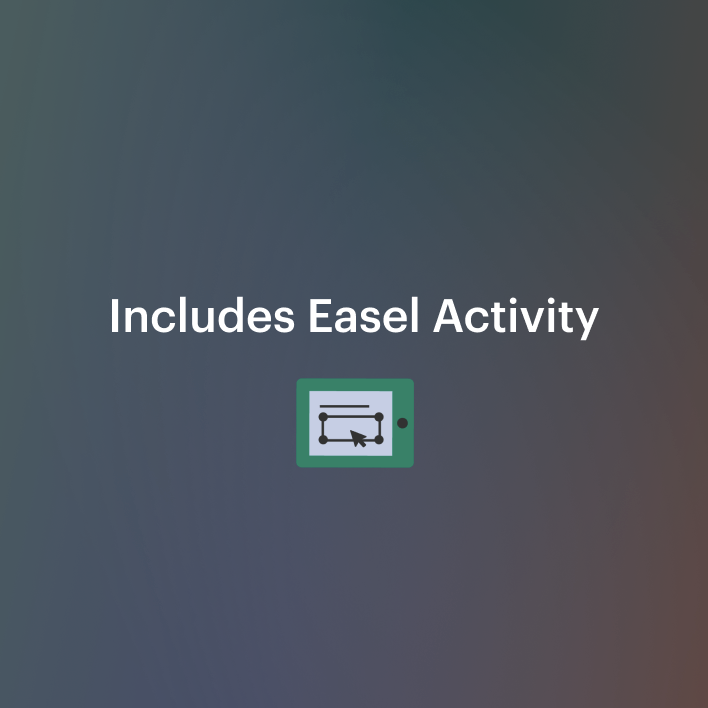DID YOU KNOW:
Seamlessly assign resources as digital activities

Learn how in 5 minutes with a tutorial resource. Try it Now

Learn More# Go Math Practice - 4th Grade Chapter 2 - Multiply by 1-Digit Numbers3rd - 5th
Subjects
Standards
Resource Type
Formats Included
• PDF
•Activity
Pages
32 pages
Easel Activity Included
This resource includes a ready-to-use interactive activity students can complete on any device. Easel by TpT is free to use! Learn more.

#### Also included in

1. What's IncludedIncluded in this pack are worksheets on all the lessons in the fourth grade Go Math series, a midchapter checkpoint practice sheet, and an end of the chapter checkpoint practice sheet for each chapter.These can be used as a quiz, formative assessment, homework, or just extra practice!
\$55.00
\$65.00
Save \$10.00
2. All Go Math!
\$88.40
\$110.50
Save \$22.10

### Description

What's Included

Included in this pack are 12 worksheets on all the lessons in the Go Math book for chapter 2, a midchapter checkpoint practice sheet, and an end of the chapter checkpoint practice sheet.

Each worksheet is 10 questions with a few exceptions. Also, all worksheets are 1 page except the end of chapter checkpoint which is 2 pages.

These can be used as a quiz, formative assessment, homework, or just extra practice!

Answer keys are included for each worksheet.

Lesson 1: Algebra • Multiplication Comparisons
Lesson 2: Algebra • Comparison Problems
Lesson 3: Multiply Tens, Hundreds, and Thousands
Lesson 4: Estimate Products
Lesson 5: Investigate • Multiply Using the Distributive Property
Lesson 6: Multiply Using Expanded Form
Lesson 7: Multiply Using Partial Products
Mid-Chapter Checkpoint (lessons 1-7)
Lesson 8: Multiply Using Mental Math
Lesson 9: Problem Solving • Multistep Multiplication Problems
Lesson 10: Multiply 2-Digit Numbers with Regrouping
Lesson 11: Multiply 3-Digit and 4-Digit Numbers with Regrouping
Lesson 12: Algebra • Solve Multistep Problems Using Equations
End of Chapter Checkpoint (lessons 8-12)

4.NBT.B.5
4.OA.A.1
4.OA.A.2
4.OA.A.3

Sample 2.1 with this freebie

Chapter 1 - Place Value, Addition, and Subtraction
Chapter 2 - Multiply by 1-Digit Numbers
Chapter 3 -
Multiply by 2-Digit Numbers

Chapter 4 - Divide by 1-Digit Numbers
Chapter 5 - Factors, Multiples, and Patterns
Chapter 6 - Fraction Equivalence and Comparison
Chapter 7 - Add and Subtract Fractions
Chapter 8 - Multiply Fractions by Whole Numbers
Chapter 9 - Relate Fractions and Decimals
Chapter 10 - Compare Fractions
Chapter 11 - Angles
Chapter 12 - Relative Size of Measurement Units
Chapter 13 - Perimeter and Area

Edit 5/22/18 - I fixed an error on 2.1
Total Pages
32 pages
Included
Teaching Duration
N/A
Report this Resource to TpT
Reported resources will be reviewed by our team. Report this resource to let us know if this resource violates TpT’s content guidelines.

### Standards

to see state-specific standards (only available in the US).
Solve multistep word problems posed with whole numbers and having whole-number answers using the four operations, including problems in which remainders must be interpreted. Represent these problems using equations with a letter standing for the unknown quantity. Assess the reasonableness of answers using mental computation and estimation strategies including rounding.
Multiply or divide to solve word problems involving multiplicative comparison, e.g., by using drawings and equations with a symbol for the unknown number to represent the problem, distinguishing multiplicative comparison from additive comparison.
Interpret a multiplication equation as a comparison, e.g., interpret 35 = 5 × 7 as a statement that 35 is 5 times as many as 7 and 7 times as many as 5. Represent verbal statements of multiplicative comparisons as multiplication equations.
Multiply a whole number of up to four digits by a one-digit whole number, and multiply two two-digit numbers, using strategies based on place value and the properties of operations. Illustrate and explain the calculation by using equations, rectangular arrays, and/or area models.